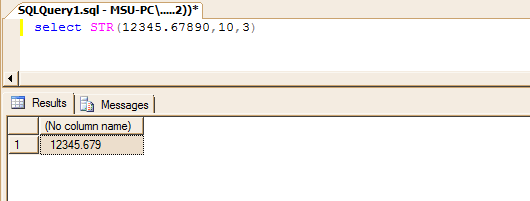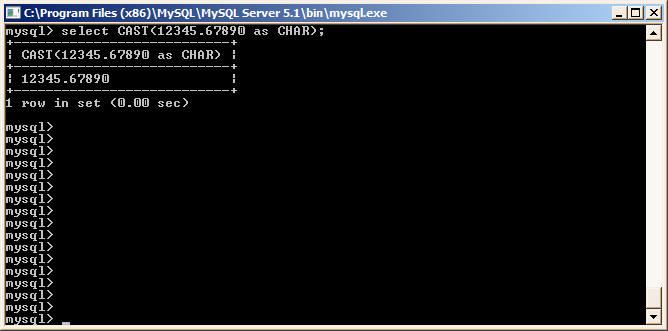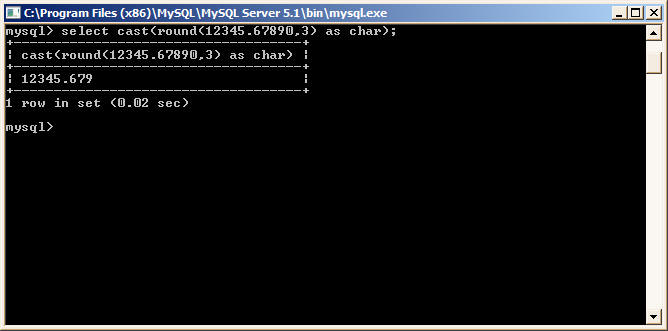﻿ MSSQL STR function equivalent in MySQL# MSSQL STR function and it's equivalent in MySQL.

The STR function in MS SQL Server is used to convert numeric values to character strings. It's syntax is given below

### Syntax

```STR ( float_expression [ , length [ , decimal ] ] )
```

#### WHERE

float_expression
Is an expression of approximate numeric (float) data type with a decimal point.

length
Is the total length. This includes decimal point, sign, digits, and spaces. The default is 10.

decimal
Is the number of places to the right of the decimal point. decimal must be less than or equal to 16. If decimal is more than 16 then the result is truncated to sixteen places to the right of the decimal point.

` `

### Example

The following query converts a number 12345.67890 to string value and also rounds the number to 3 decimal places

```select STR(12345.67890,12,3)
--------------------------
12345.679```
``

## MySQL

In MySQL the same functionality can be achieved by using CAST function. The CAST function will convert numeric value
to character string. If you also want to round the number to N decimal places as in MSSQL then you have to use ROUND
function also.

### Example

```mysql> select CAST(12345.67890 as CHAR);
+---------------------------+
| CAST(12345.67890 as CHAR) |
+---------------------------+
| 12345.67890 		    |
+---------------------------+
1 row in set (0.00 sec)
```
``
` `
```mysql> select cast(round(12345.67890,3) as char);
+------------------------------------+
| cast(round(12345.67890,3) as char) |
+------------------------------------+
| 12345.679 |
+------------------------------------+
1 row in set (0.02 sec)

mysql>```
``
` `

Back to Converting Functions from MSSQL to MySQL

` `